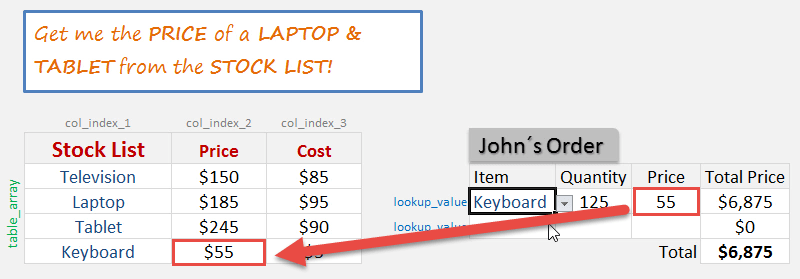What does it do?

Searches for a value in the first column of a table array and returns a value in the same row from another column (to the right) in the table array.

Formula breakdown:

=VLOOKUP(lookup_value, table_array, col_index_num, [range_lookup])

What it means:

=VLOOKUP(this value, in this list, and get me value in this column, Exact Match/FALSE/0])The VLOOKUP function in Excel can become interactive and more powerful when applying a Data Validation (drop down menu/list) as the Lookup_Value.  So as you change your selection from the drop down list, the VLOOKUP value also changes.

Click to learn how to insert a drop down menu with Data ValidationSee how easy it is to apply this with a quick VLOOKUP example below.

STEP 1: Go to Data > Data Validation.STEP 2: Select List in the Allow dropdown

For the Source, ensure that it has the 4 Stock List values selected. Click OK.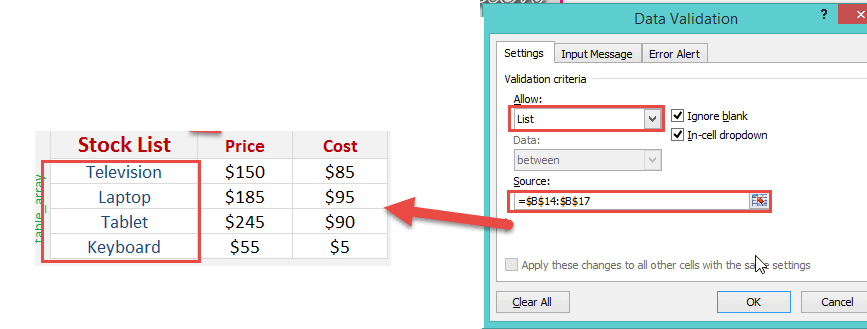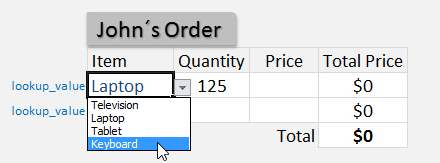STEP 3: We need to enter the Vlookup function:

## +VLOOKUP(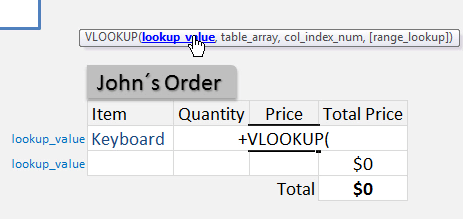The Vlookup arguments:

## lookup_value

What are we looking for?

Reference the cell that contains the text or value:

## =VLOOKUP(G15,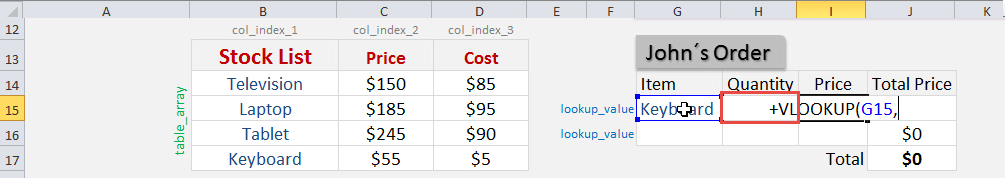## table_array

From which list are we doing a lookup on?

Place in the cell range of the Stock List:

## +VLOOKUP(G15, \$B\$14:\$D\$17,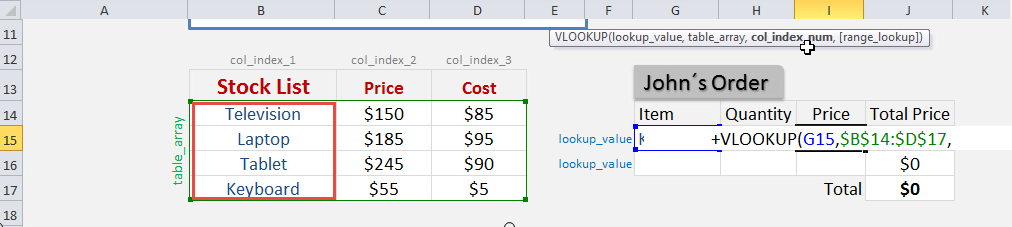## col_index_num

From which column do we want to retrieve the value?

We want to retrieve the Price which is the SECOND column from our table array:

## +VLOOKUP(G15, \$B\$14:\$D\$17, 2,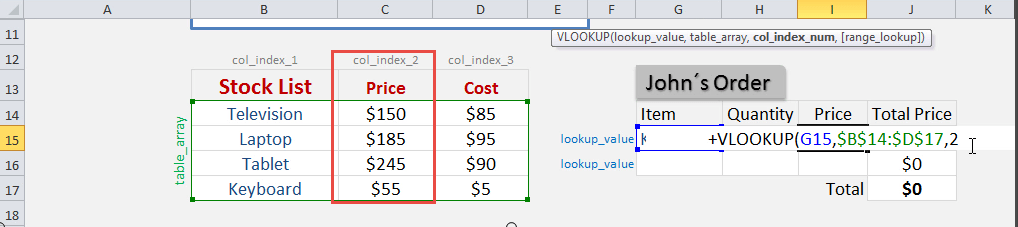## [range_lookup]

Do we want an exact match?

Place in FALSE to signify that we want an exact match:

## +VLOOKUP(G15, \$B\$14:\$D\$17, 2, FALSE)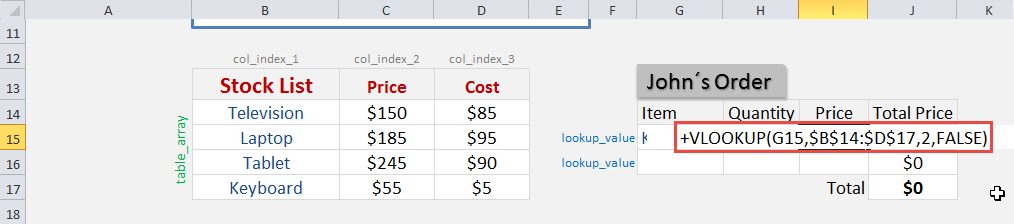The price now dynamically changes based on your selection: